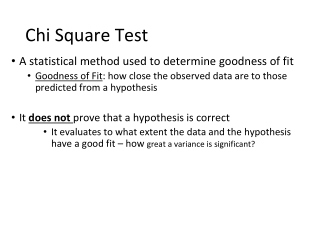DownloadDownload PresentationChi Square Test

# Chi Square Test

Download Presentation## Chi Square Test

- - - - - - - - - - - - - - - - - - - - - - - - - - - E N D - - - - - - - - - - - - - - - - - - - - - - - - - - -
##### Presentation Transcript

1. Chi Square Test • A statistical method used to determine goodness of fit • Goodness of Fit: how close the observed data are to those predicted from a hypothesis • It does not prove that a hypothesis is correct • It evaluates to what extent the data and the hypothesis have a good fit – how great a variance is significant?

2. Chi Square Test • Formula • o = observed data in each category • e = observed data in each category based on the experimenter’s hypothesis • ∑ = Sum of the calculations for each category (o – e)2 χ2 = ∑ e

3. Chi Square Test • Null Hypothesis: hypothesis that states there will be no difference between expected and observed data • You wonder if musicians have faster reaction times than athletes • Null Hypothesis – there is no difference between the two • Of 100 offspring, there will be a ratio of ¾ dominant (red) to ¼ recessive (white)

4. Suppose a cross between two pea plants yields a population of 880 plants, 639 with green seeds and 241 with yellow seeds. You are asked to propose the genotypes of the parents. Your hypothesis is that the allele for green is dominant to yellow and both parents are heterozygous. If your hypothesis is true, then the predicted ratio of offspring from the cross would be 3:1 as predicted from the results of a Punnett square. Is it?

5. Chi Square Test • Example: Observed Expected* Frequency Frequency (o) (e) red flowers 73 75 white flowers 27 25 *The expected frequency depends upon the hypothesis.

6. Chi Square Test (o - e )2 o – e (o - e)2 e red flowers -2 4 0.053 white flowers 2 4 0.16 (o – e)2 χ2 = ∑ e = 0.053 + 0.16 = 0.213 Divide by 4/75 for RED = 0.053 and 4/ 25 for white= 0.16 add together for total population= 0.213

7. Chi Square Test • Degrees of Freedom: critical factor in chi square, essentially the number of independent random variables involved. • Degrees of freedom is simply the number of classes of offspring minus 1 (d.f. = n-1). • For our example, there are 2 classes of offspring: red and white. Thus, degrees of freedom (d.f.) = 2 -1 = 1.

8. Chi Square Test • Critical values for chi-square are found on tables, sorted by degrees of freedom and probability levels. • A p-value gives us the probability that the null hypothesis (expected=observed) is correct. Alwaysuse p = 0.05.

9. Chi Square Test Your value χ2 = 0.213 Do you accept or reject the null hypothesis?

10. Chi Square Test • If chi-square value is greater than the critical value from the table, “reject the null hypothesis.” • If chi-square value is less than the critical value, you “fail to reject” the null hypothesis (that is, you accept that your genetic theory about the expected ratio is correct).